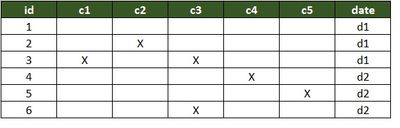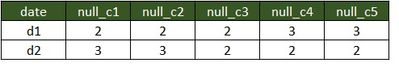# Support Questions

Announcements
Celebrating as our community reaches 100,000 members! Thank you!

## HIVE : counting null values based on group byarunak
Super Collaborator

Hello All,

I am trying to group all records for a table by "date" which is also a column. I need to count the number of null values for each column in the table grouped by date.

All the columns are of numeric type double/int.

Below is a sample input/output requirementSimple select query that helps accomplish this requirement is select date,count(*) as c1_null from t1 where c1 is null group by date

I chain this select pattern for every c'i' columns.

This works fine only if every value for a given column is null and returns an empty result set if at least one column is non null.

What is a way to accomplish this?

Any help appreciated.

Thanks -arun

1 ACCEPTED SOLUTIONamcbarnett
Guru
```WITH t1nulltest AS
( select date_column
,SUM(IF(c1 IS NULL,1,0)) OVER (PARTITION BY date_column) as c1null
,SUM(IF(c2 IS NULL,1,0)) OVER (PARTITION BY date_column) as c2null
,SUM(IF(c3 IS NULL,1,0)) OVER (PARTITION BY date_column) as c3null
,SUM(IF(c4 IS NULL,1,0)) OVER (PARTITION BY date_column) as c4null
,SUM(IF(c5 IS NULL,1,0)) OVER (PARTITION BY date_column) as c5null
,row_number() OVER (PARTITION BY date_column) as rowno
from t1)
select
date_column, c1null, c2null,c3null,c4null,c5null from t1nulltest
where rowno =1;```
5 REPLIES 5arunak
Super Collaborator

Sample create and insert as below -

```create table t1(date_column string, c1 int, c2 int, c3 int, c4 int, c5 int);

insert into t1 values("d1",1,1,1,1,1);
insert into t1 (date_column,c1) values ("d1",1);
insert into t1 (date_column,c1) values ("d2",1);
insert into t1 values("d2",1,1,1,1,1);```

I was expecting the below query to return 0 for d1 and d2, unfortunately got an empty result set.

```select count(*) from t1 where c1 is null group by date_column;
```amcbarnett
Guru
```WITH t1nulltest AS
( select date_column
,SUM(IF(c1 IS NULL,1,0)) OVER (PARTITION BY date_column) as c1null
,SUM(IF(c2 IS NULL,1,0)) OVER (PARTITION BY date_column) as c2null
,SUM(IF(c3 IS NULL,1,0)) OVER (PARTITION BY date_column) as c3null
,SUM(IF(c4 IS NULL,1,0)) OVER (PARTITION BY date_column) as c4null
,SUM(IF(c5 IS NULL,1,0)) OVER (PARTITION BY date_column) as c5null
,row_number() OVER (PARTITION BY date_column) as rowno
from t1)
select
date_column, c1null, c2null,c3null,c4null,c5null from t1nulltest
where rowno =1;```arunak
Super Collaborator

Thanks @Ancil McBarnett. Appreciate it.vgargContributor

You can also achieve this by using following query:

```SELECT date_column,
count(*)-count(c1) as null_c1,
count(*)-count(c2) as null_c2,
count(*)-count(c3) as null_c3,
count(*)-count(c4) as null_c4
FROM t1
GROUP BY date_column;
```shetty_vikas
New Contributor

@amcbarnett : i am trying to aggregate a data using "state,count( distinct val ) group by state " but want just the "Not Null"

Val - String datatype